# Basic MIPS implementation

16 de Feb de 2017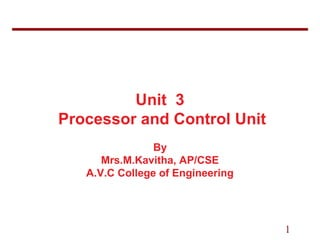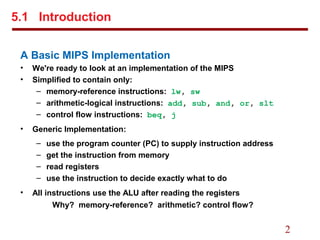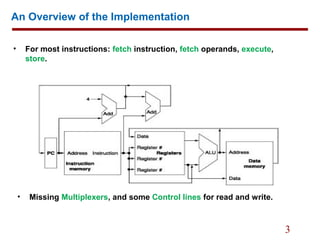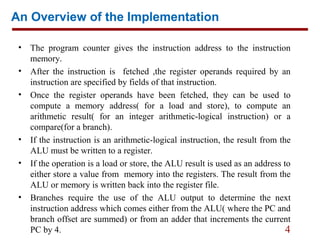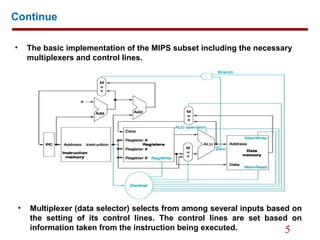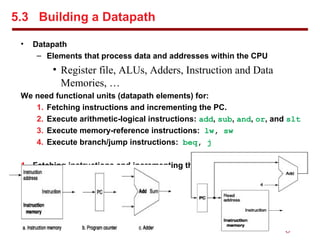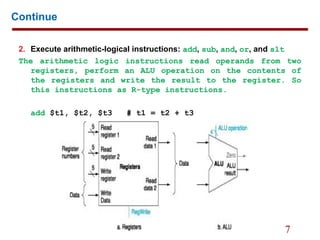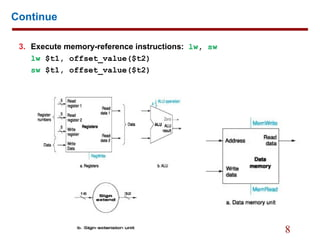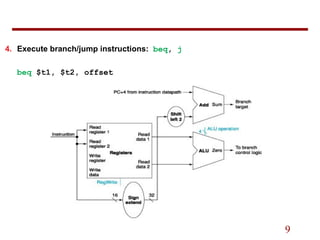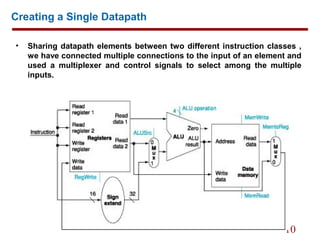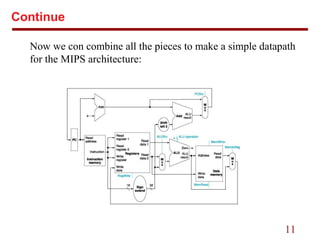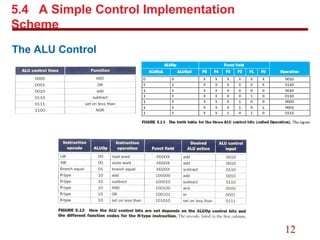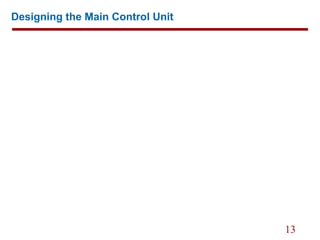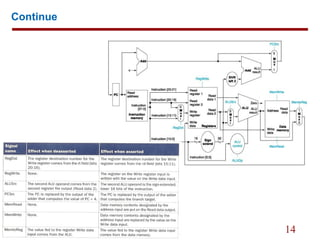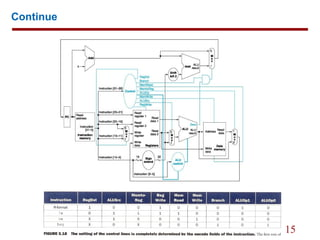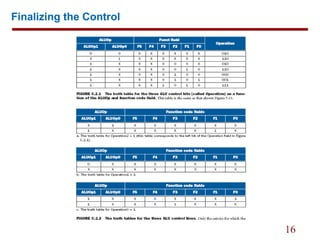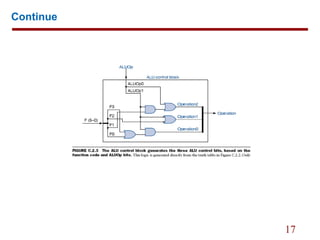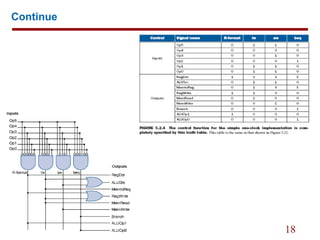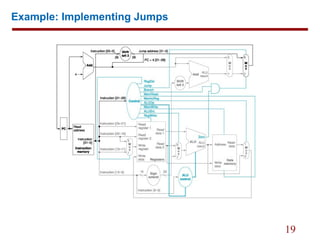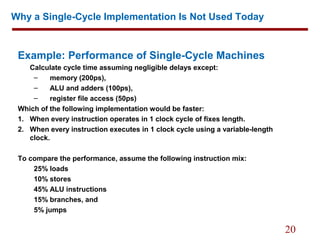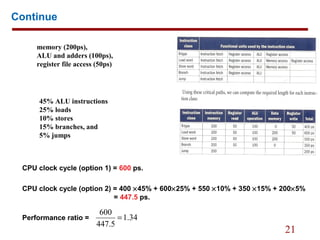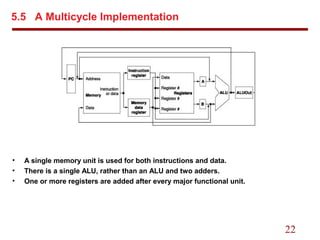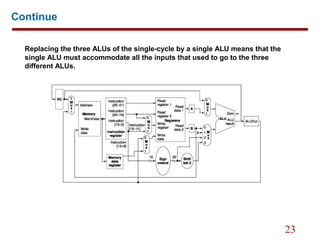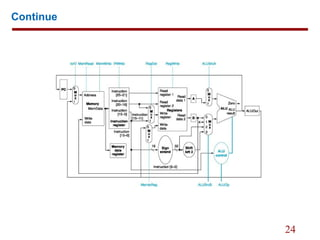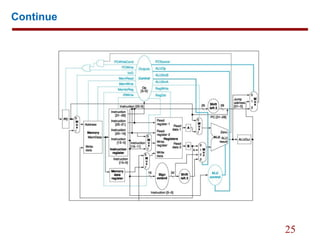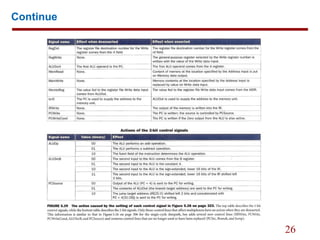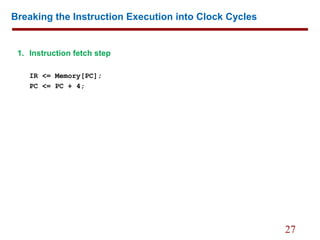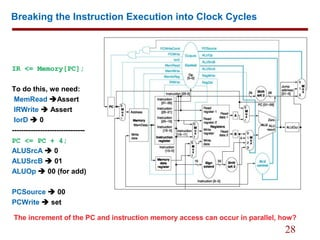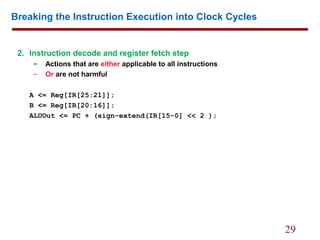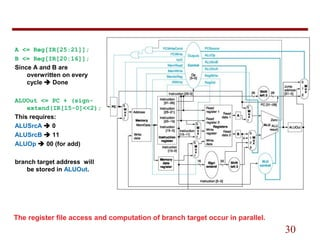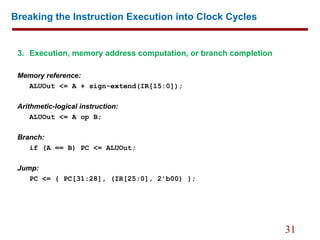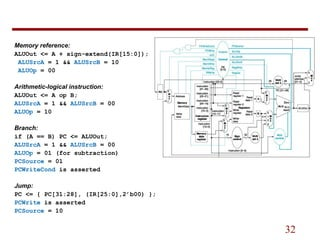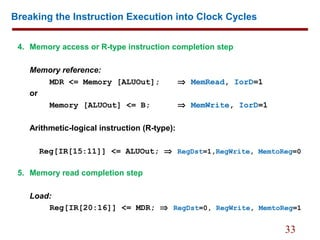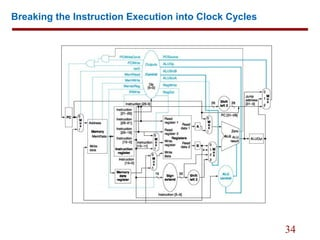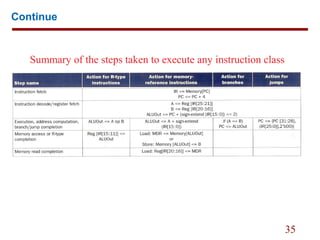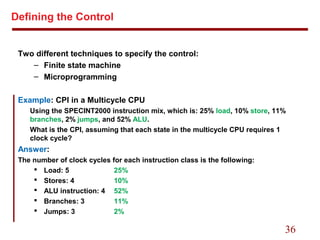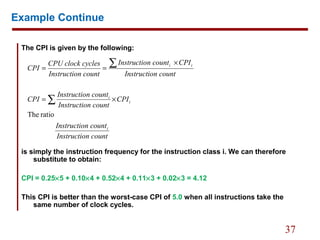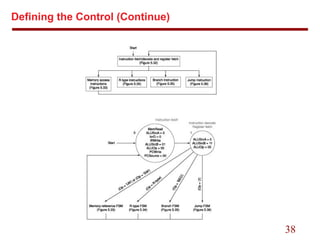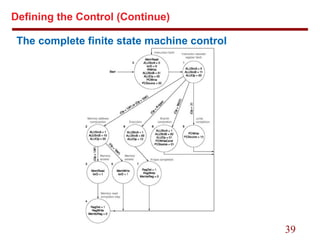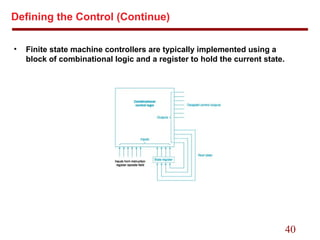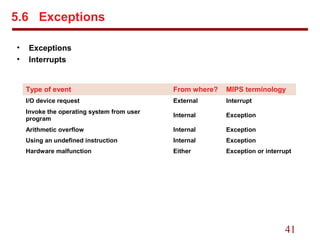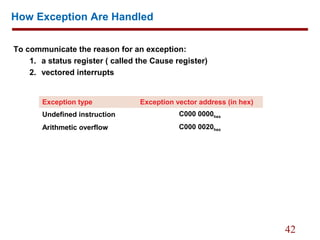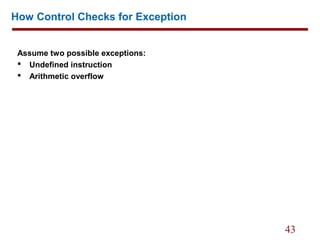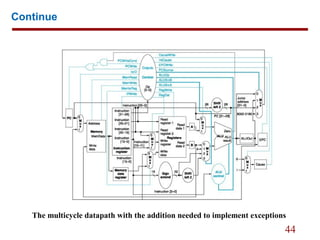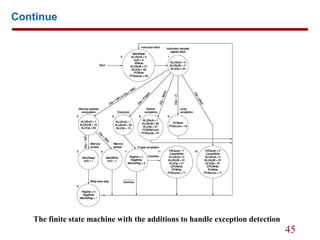1 de 45

### Basic MIPS implementation

• 1. 1 Unit 3 Processor and Control Unit By Mrs.M.Kavitha, AP/CSE A.V.C College of Engineering
• 2. 2 A Basic MIPS Implementation • We're ready to look at an implementation of the MIPS • Simplified to contain only: – memory-reference instructions: lw, sw – arithmetic-logical instructions: add, sub, and, or, slt – control flow instructions: beq, j • Generic Implementation: – use the program counter (PC) to supply instruction address – get the instruction from memory – read registers – use the instruction to decide exactly what to do • All instructions use the ALU after reading the registers Why? memory-reference? arithmetic? control flow? 5.1 Introduction
• 3. 3 An Overview of the Implementation • For most instructions: fetch instruction, fetch operands, execute, store. • Missing Multiplexers, and some Control lines for read and write.
• 4. 4 An Overview of the Implementation • The program counter gives the instruction address to the instruction memory. • After the instruction is fetched ,the register operands required by an instruction are specified by fields of that instruction. • Once the register operands have been fetched, they can be used to compute a memory address( for a load and store), to compute an arithmetic result( for an integer arithmetic-logical instruction) or a compare(for a branch). • If the instruction is an arithmetic-logical instruction, the result from the ALU must be written to a register. • If the operation is a load or store, the ALU result is used as an address to either store a value from memory into the registers. The result from the ALU or memory is written back into the register file. • Branches require the use of the ALU output to determine the next instruction address which comes either from the ALU( where the PC and branch offset are summed) or from an adder that increments the current PC by 4.
• 5. 5 Continue • The basic implementation of the MIPS subset including the necessary multiplexers and control lines. • Multiplexer (data selector) selects from among several inputs based on the setting of its control lines. The control lines are set based on information taken from the instruction being executed.
• 6. 6 5.3 Building a Datapath • Datapath – Elements that process data and addresses within the CPU • Register file, ALUs, Adders, Instruction and Data Memories, … We need functional units (datapath elements) for: 1. Fetching instructions and incrementing the PC. 2. Execute arithmetic-logical instructions: add, sub, and, or, and slt 3. Execute memory-reference instructions: lw, sw 4. Execute branch/jump instructions: beq, j 1. Fetching instructions and incrementing the PC.
• 7. 7 Continue 2. Execute arithmetic-logical instructions: add, sub, and, or, and slt The arithmetic logic instructions read operands from two registers, perform an ALU operation on the contents of the registers and write the result to the register. So this instructions as R-type instructions. add \$t1, \$t2, \$t3 # t1 = t2 + t3
• 8. 8 Continue 3. Execute memory-reference instructions: lw, sw lw \$t1, offset_value(\$t2) sw \$t1, offset_value(\$t2)
• 9. 9 4. Execute branch/jump instructions: beq, j beq \$t1, \$t2, offset
• 10. 10 Creating a Single Datapath • Sharing datapath elements between two different instruction classes , we have connected multiple connections to the input of an element and used a multiplexer and control signals to select among the multiple inputs.
• 11. 11 Continue Now we con combine all the pieces to make a simple datapath for the MIPS architecture:
• 12. 12 5.4 A Simple Control Implementation Scheme The ALU Control
• 13. 13 Designing the Main Control Unit
• 14. 14 Continue
• 15. 15 Continue
• 16. 16 Finalizing the Control
• 17. 17 Continue
• 18. 18 Continue
• 19. 19 Example: Implementing Jumps
• 20. 20 Why a Single-Cycle Implementation Is Not Used Today Example: Performance of Single-Cycle Machines Calculate cycle time assuming negligible delays except: – memory (200ps), – ALU and adders (100ps), – register file access (50ps) Which of the following implementation would be faster: 1. When every instruction operates in 1 clock cycle of fixes length. 2. When every instruction executes in 1 clock cycle using a variable-length clock. To compare the performance, assume the following instruction mix: 25% loads 10% stores 45% ALU instructions 15% branches, and 5% jumps
• 21. 21 Continue CPU clock cycle (option 1) = 600 ps. CPU clock cycle (option 2) = 400 ×45% + 600×25% + 550 ×10% + 350 ×15% + 200×5% = 447.5 ps. Performance ratio = 34.1 5.447 600 = 45% ALU instructions 25% loads 10% stores 15% branches, and 5% jumps memory (200ps), ALU and adders (100ps), register file access (50ps)
• 22. 22 5.5 A Multicycle Implementation • A single memory unit is used for both instructions and data. • There is a single ALU, rather than an ALU and two adders. • One or more registers are added after every major functional unit.
• 23. 23 Continue Replacing the three ALUs of the single-cycle by a single ALU means that the single ALU must accommodate all the inputs that used to go to the three different ALUs.
• 24. 24 Continue
• 25. 25 Continue
• 26. 26 Continue
• 27. 27 Breaking the Instruction Execution into Clock Cycles 1. Instruction fetch step IR <= Memory[PC]; PC <= PC + 4;
• 28. 28 Breaking the Instruction Execution into Clock Cycles IR <= Memory[PC]; To do this, we need: MemRead Assert IRWrite  Assert IorD  0 ------------------------------- PC <= PC + 4; ALUSrcA  0 ALUSrcB  01 ALUOp  00 (for add) PCSource  00 PCWrite  set The increment of the PC and instruction memory access can occur in parallel, how?
• 29. 29 Breaking the Instruction Execution into Clock Cycles 2. Instruction decode and register fetch step – Actions that are either applicable to all instructions – Or are not harmful A <= Reg[IR[25:21]]; B <= Reg[IR[20:16]]; ALUOut <= PC + (sign-extend(IR[15-0] << 2 );
• 30. 30 A <= Reg[IR[25:21]]; B <= Reg[IR[20:16]]; Since A and B are overwritten on every cycle  Done ALUOut <= PC + (sign- extend(IR[15-0]<<2); This requires: ALUSrcA  0 ALUSrcB  11 ALUOp  00 (for add) branch target address will be stored in ALUOut. The register file access and computation of branch target occur in parallel.
• 31. 31 Breaking the Instruction Execution into Clock Cycles 3. Execution, memory address computation, or branch completion Memory reference: ALUOut <= A + sign-extend(IR[15:0]); Arithmetic-logical instruction: ALUOut <= A op B; Branch: if (A == B) PC <= ALUOut; Jump: PC <= { PC[31:28], (IR[25:0], 2’b00) };
• 32. 32 Memory reference: ALUOut <= A + sign-extend(IR[15:0]); ALUSrcA = 1 && ALUSrcB = 10 ALUOp = 00 Arithmetic-logical instruction: ALUOut <= A op B; ALUSrcA = 1 && ALUSrcB = 00 ALUOp = 10 Branch: if (A == B) PC <= ALUOut; ALUSrcA = 1 && ALUSrcB = 00 ALUOp = 01 (for subtraction) PCSource = 01 PCWriteCond is asserted Jump: PC <= { PC[31:28], (IR[25:0],2’b00) }; PCWrite is asserted PCSource = 10
• 33. 33 Breaking the Instruction Execution into Clock Cycles 4. Memory access or R-type instruction completion step Memory reference: MDR <= Memory [ALUOut]; ⇒ MemRead, IorD=1 or Memory [ALUOut] <= B; ⇒ MemWrite, IorD=1 Arithmetic-logical instruction (R-type): Reg[IR[15:11]] <= ALUOut; ⇒ RegDst=1,RegWrite, MemtoReg=0 5. Memory read completion step Load: Reg[IR[20:16]] <= MDR; ⇒ RegDst=0, RegWrite, MemtoReg=1
• 34. 34 Breaking the Instruction Execution into Clock Cycles
• 35. 35 Continue Summary of the steps taken to execute any instruction class
• 36. 36 Defining the Control Two different techniques to specify the control: – Finite state machine – Microprogramming Example: CPI in a Multicycle CPU Using the SPECINT2000 instruction mix, which is: 25% load, 10% store, 11% branches, 2% jumps, and 52% ALU. What is the CPI, assuming that each state in the multicycle CPU requires 1 clock cycle? Answer: The number of clock cycles for each instruction class is the following:  Load: 5 25%  Stores: 4 10%  ALU instruction: 4 52%  Branches: 3 11%  Jumps: 3 2%
• 37. 37 Example Continue The CPI is given by the following: is simply the instruction frequency for the instruction class i. We can therefore substitute to obtain: CPI = 0.25×5 + 0.10×4 + 0.52×4 + 0.11×3 + 0.02×3 = 4.12 This CPI is better than the worst-case CPI of 5.0 when all instructions take the same number of clock cycles. n countInstructio n countInstructio CPI n countInstructio n countInstructio CPI n countInstructio CPIn countInstructio n countInstructio cyclesCPU clock CPI i i i ii ratioThe ∑ ∑ ×= × ==
• 38. 38 Defining the Control (Continue)
• 39. 39 Defining the Control (Continue) The complete finite state machine control
• 40. 40 Defining the Control (Continue) • Finite state machine controllers are typically implemented using a block of combinational logic and a register to hold the current state.
• 41. 41 5.6 Exceptions • Exceptions • Interrupts Type of event From where? MIPS terminology I/O device request External Interrupt Invoke the operating system from user program Internal Exception Arithmetic overflow Internal Exception Using an undefined instruction Internal Exception Hardware malfunction Either Exception or interrupt
• 42. 42 How Exception Are Handled To communicate the reason for an exception: 1. a status register ( called the Cause register) 2. vectored interrupts Exception type Exception vector address (in hex) Undefined instruction C000 0000hex Arithmetic overflow C000 0020hex
• 43. 43 How Control Checks for Exception Assume two possible exceptions:  Undefined instruction  Arithmetic overflow
• 44. 44 Continue The multicycle datapath with the addition needed to implement exceptions
• 45. 45 Continue The finite state machine with the additions to handle exception detection